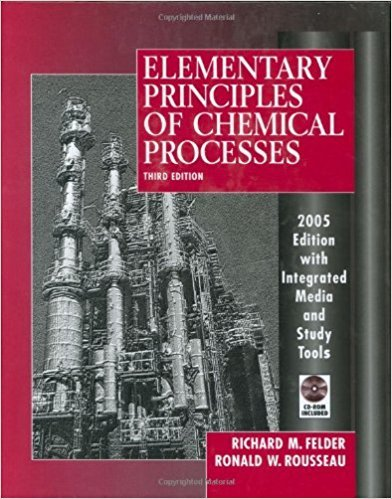×
Get Full Access to Elementary Principles Of Chemical Processes - 3 Edition - Chapter 6 - Problem 6.9
Get Full Access to Elementary Principles Of Chemical Processes - 3 Edition - Chapter 6 - Problem 6.9

×

# Liquid methyl ethyl ketone (MEK) is introduced into aISBN: 9780471687573 143

## Solution for problem 6.9 Chapter 6

Elementary Principles of Chemical Processes | 3rd Edition

• Textbook Solutions
• 2901 Step-by-step solutions solved by professors and subject experts
• Get 24/7 help from StudySoup virtual teaching assistantsElementary Principles of Chemical Processes | 3rd Edition

4 5 1 236 Reviews
25
5
Problem 6.9

Liquid methyl ethyl ketone (MEK) is introduced into a vessel containing air. The system temperature is increased to 55C, and the vessel contents reach equilibrium with some MEK remaining in the liquid state. The equilibrium pressure is 1200 mm Hg. (a) Use the Gibbs phase rule to determine how many degrees of freedom exist for the system at equilibrium. State the meaning of your result in your own words. (b) Mixtures of MEK vapor and air that contain between 1.8 mole% MEK and 11.5 mole% MEK can ignite and burn explosively if exposed to a flame or spark. Determine whether or not the given vessel constitutes an explosion hazard.

Step-by-Step Solution:

Problem 6.9Liquid methyl ethyl ketone (MEK) is introduced into a vessel containing air. The systemtemperature is increased to 55°C, and the vessel contents reach equilibrium with some MEKremaining in the liquid state. The equilibrium pressure is 1200 mm Hg. (a) Use the Gibbs phase rule to determine how many degrees of freedom exist for the system at equilibrium. State the meaning of your result in your own words. (b) Mixtures of MEK vapor and air that contain between 1.8 mole% MEK and 11.5 mole% MEK can ignite and burn explosively if exposed to a flame or spark. Determine whether or not the given vessel constitutes an explosion hazard. Step-by-step solution Step 1 of 3 ^Represent the system of methyl ethyl ketone liquid in vessel of air as shown below:Air T = 55°C MEK (l) P = 1200 mm Hg(a)The Gibbs Phase Rule is given below: DF = 2 + c Here, is number of phases in a system at equilibrium, c is number of chemical species, andDF is degrees of freedom.In the system, there are two chemical species, dry air and MEK, therefore c = 2. It is a twophase system, liquid and vapor, therefore = 2.Calculate the degrees of freedom, DF.\n DF = 2 + c = 2 + 2 - 2 = 2The system has 2 degrees of freedom. This means that if two intensive variables, such astemperature and pressure, are fixed then other intensive variables can be calculated becausethey are not independent.

Step 2 of 3

Step 3 of 3

##### ISBN: 9780471687573

Unlock Textbook Solution